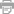# Where Is The Line Lyrics

## Bjá¶rk

### Non-album songs

Lyrics to Where Is The Line
Where is the line with you
Where is the -- line - with you
Where is the line with you
Where is the -- line - with you

My purse wide open
I see the crime and cash into
Accounts
Everywhere

Where is the line with you
Where is the line -- with you
Where is the -- line - with you
Where is the -- line with you

I want to be flexible
I want to go out of my way for you
But enough is enough

Where is the line with you
Where is the -- line with you
Where is the line with you
Where is the -- line - with you

I am elastic
I want to
Go out of my way for you
I want to
Help

Where is the line with you
Where is the line - with you
Where is the -- line - with you
Where is the -- line - with you

I want to
Have
Capacity
For you and be elastic
Love
To be elastic
E-la-stic for

Where is the line with you
Where is the -- line with you
Where is the line with you
Where is the line with you
Where is the line - with you
Where -- is the line with you

I'm elastic for you
But enough is enough
Songwriters: GUDMUNDSDOTTIR, BJORK
Publisher: Lyrics © Universal Music Publishing Group
•Print